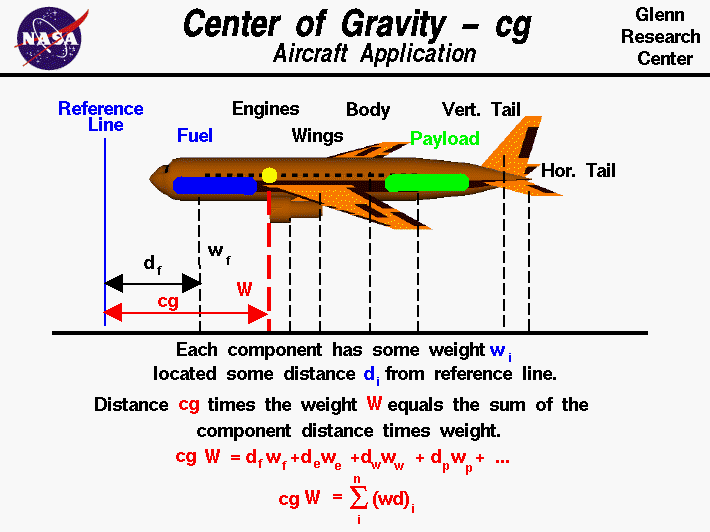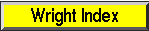+ Text Only Site
+ Non-Flash Version
+ Contact GlennAn airplane in flight can be maneuvered by the pilot using the aerodynamic control surfaces; the elevator, rudder, or ailerons. As the control surfaces change the amount of force that each surface generates, the aircraft rotates about a point called the center of gravity. The center of gravity is the average location of the weight of the aircraft. The weight is actually distributed throughout the airplane, and for some problems it is important to know the distribution. But for total aircraft maneuvering, we need to be concerned with only the total weight and the location of the center of gravity. How do engineers determine the location of the center of gravity for an airplane which they are designing? An airplane is a combination of many parts; the wings, engines, fuselage, and tail, plus the payload and the fuel. Each part has a weight associated with it which the engineer can estimate, or calculate, using Newton's weight equation: w = m * g where w is the weight, m is the mass, and g is the gravitational constant which is 32.2 ft/square sec in English units and 9.8 meters/square sec in metric units. To determine the center of gravity cg, we choose a reference location, or reference line. The cg is determined relative to this reference location. The total weight of the aircraft is simply the sum of all the individual weights of the components. Since the center of gravity is an average location of the weight, we can say that the weight of the entire aircraft W times the location cg of the center of gravity is equal to the sum of the weight w of each component times the distance d of that component from the reference location: W * cg = [w * d](fuselage) + [w * d](wing) + [w * d](engines) + ... The center of gravity is the mass-weighted average of the component locations. We can generalize the technique discussed above. If we had a total of "n" discrete components, the center of gravity cg of the aircraft times the weight W of the aircraft would be the sum of the individual i component weight times the distance d from the reference line (w * d) with the index i going from 1 to n. Mathematicians use the greek letter sigma to denote this addition. (Sigma is a zig-zag symbol with the index designation being placed below the bottom bar, the total number of additions placed over the top bar, and the variable to be summed placed to the right of the sigma with each component designated by the index.) W * cg = SUM(i=1 to i=n) [w * d]i This equation says that the center of gravity times the sum of "n" parts' weight is equal to the sum of "n" parts' weight times their distance. The discrete equation works for "n" discrete parts. Activities:Guided ToursAircraft Weight:Fuselage:Navigation ..Beginner's Guide Home Page+ Inspector General Hotline + Equal Employment Opportunity Data Posted Pursuant to the No Fear Act + Budgets, Strategic Plans and Accountability Reports + Freedom of Information Act + The President's Management Agenda + NASA Privacy Statement, Disclaimer, and Accessibility CertificationEditor: Nancy Hall NASA Official: Nancy Hall Last Updated: May 13 2021 + Contact Glenn